# Evaluation

## Comparison of LES results with experiments

 Figure 4: Skin-friction and pressure coefficients at the wall. Symbols: experiment [‌31], solid line: simulation.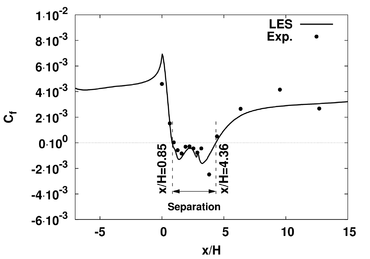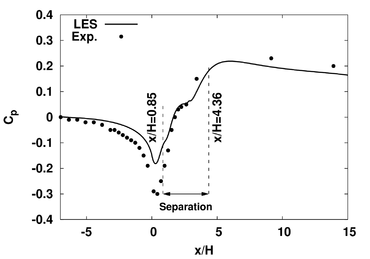Figure 5: Streamwise ($U$) and wall-normal ($V$) velocity profiles. Symbols: experiment [‌31], solid line: simulation. The dashed line delimitates the region of negative average streamwise velocity.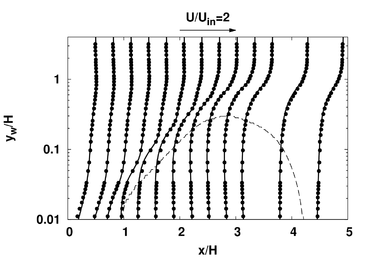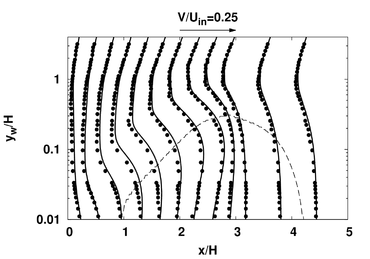Comparisons of the simulation results with the experimental measurements of Zhang and Zhong [‌31] are shown in Figs. 4 – 6. Only results obtained with the fine mesh (ie. corresponding to the data provided), are shown here. Comparisons with the coarse mesh results are given in Lardeau and Leschziner [‌13]. General agreement of the profiles is very good, both for mean flow velocity Fig. 5 and for the three components of the Reynolds stress tensor (Fig. 6). The major discrepancies are found on the wall-measurements: the skin-friction coefficient ${C_{f}}$is slightly overestimated close to the separation, with a large peak of ${C_{f}}$not present in the experimental results. The experimental skin friction data should be taken with care, as they were computed from the measured velocity field (bound to greater error in the near-wall region) rather than from direct measurements at the wall.

 Figure 6: Streamwise (${\overline {uu}}$), wall-normal (${\overline {vv}}$) and shear-stress (${-{\overline {uv}}}$) profiles. Symbols: experiment [‌31], solid line: simulation. The dashed line delimitates the region of negative streamwise velocity.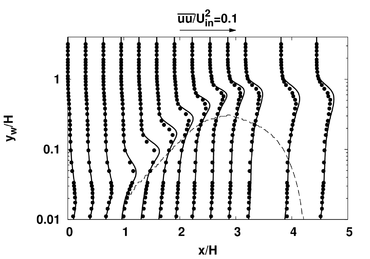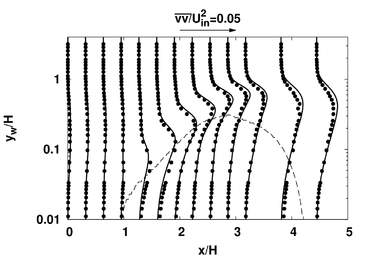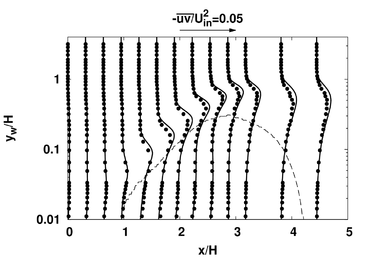## Comparison of RANS results with LES results and experiments

Results obtained with the four RANS models are shown in Figs. 7 – 11}. Only four quantities (wall shear stress, pressure coefficient, streamwise velocity and turbulent kinetic energy) are shown, but other parameters can of course be compared, such as dissipation or Reynolds-stress if using second-moment closure.

 Figure 7: Comparison of RANS results with simulation data for (left column) wall shear stress and (right column) pressure coefficient ${C_{p}}$.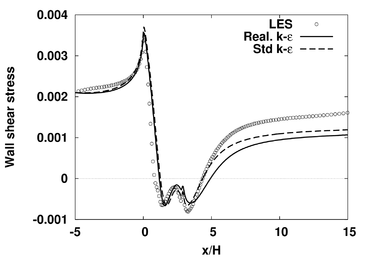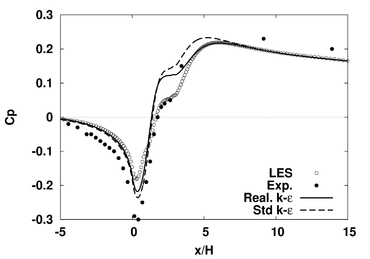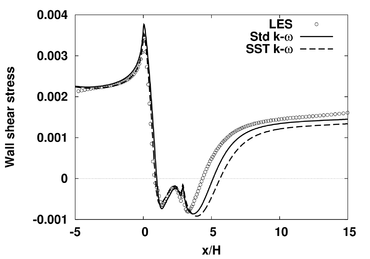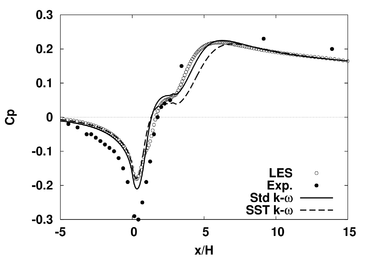Model ${x_{sep}/H}$${x_{att}/H}$${L_{sep}}$$\Delta$(%) Inflow condition
LES 0.83 4.36 3.53 n.a. n.a.
Standard ${k-\varepsilon }$1.25 4.36 3.11 12 ${\varepsilon _{\mathrm {inflow} }=0.001}$Realizable ${k-\varepsilon }$1.19 4.905 3.71 5 ${\varepsilon _{\mathrm {inflow} }=0.001}$Standard ${k-\omega }$0.98 5.12 4.14 17 ${\omega _{\mathrm {inflow} }=0.1U_{in}/H}$SST ${k-\omega }$0.87 5.62 4.75 34.5 ${\omega _{\mathrm {inflow} }=0.1U_{in}/H}$Table 2: Separation and reattachment position for all 4 RANS calculations compared to LES results. Specific inflow condition are also given. Error is given by ${\Delta =100\left|{\frac {L_{sep,LES}-L_{sep,model}}{L_{sep,LES}}}\right|}$.

The streamwise evolution of the wall shear stress ${\tau _{w,x}}$and the pressure coefficient are shown in Fig. 7. As mentioned in the Test Case section, the inflow condition was designed to match the behaviour of the wall shear stress up to ${x/H=0}$(including the peak near ${x/H=0}$), and this is indeed the case for all the models. The difference between the models occurs just downstream of the peak: both ${k-\varepsilon }$models return a slightly too late separation, while the two ${k-\omega }$models are in much better agreement with the LES data, up to ${x/H=3}$. Inside the separated region, all four models predict more or less the same wall shear stress. The only model to return a correct reattachment point is the standard ${k-\varepsilon }$model, but the recovery region downstream (from ${x/H=7}$) is much better predicted by both ${k-\omega }$models. Corresponding values for the separation and the reattachment points are given in table 2. These observations are also valid for the pressure coefficient ${C_{p}}$: before separation, the flow is fairly well predicted by all four models, compared to the LES data, with the same difference (too little flow acceleration) compare to the experimental data, included in the plot. Differences between the models only arise close to the separation point, with a slightly larger flow acceleration predicted by the ${k-\varepsilon }$models. Overall, any of the two ${k-\omega }$models return a much better qualitative picture than the ${k-\varepsilon }$formulations. However, table 2 shows that the separation bubble is much larger (up to 35%) than in the LES. Other, more advanced, ${k-\varepsilon }$models were found to give better results for this test case, but once again, the objective of this document is to provide guidelines, not an assessment of model capabilities.

 Figure 8: Comparison of velocity profiles with simulation data for the two ${k-\varepsilon }$models. Profiles are shifted by ${0.2U_{in}}$for consecutive downstream positions, for clarity. Also, semi-log scaling is used to highlight the differences in the results when the flow is attached, i.e. for (a) and (d) (also in Figs. 9–11).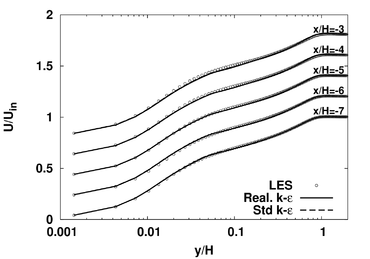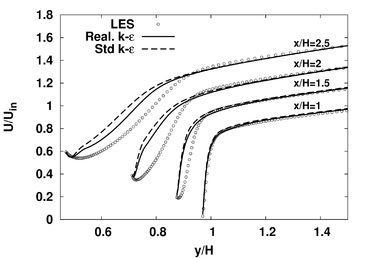(a) (b)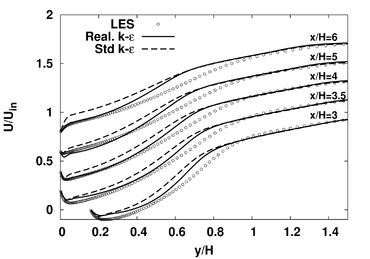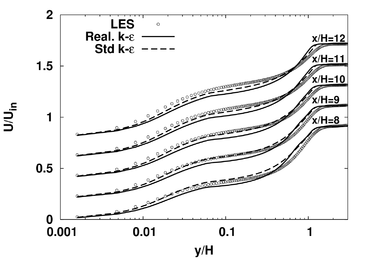(c) (d)

The differences between the different models in predicting wall data can be better understood by comparing profiles of the streamwise velocity component (Figs. 8 and 10) and the turbulent kinetic energy (Figs. 9 and 11) at different locations upstream of the bump, in the separated region and in the recovery region. The two sets of models (${k-\varepsilon }$and ${k-\omega }$) have clear differences, especially close to the inlet (from ${x/H=-7}$to ${x/H=-3}$). For ${k-\varepsilon }$, velocity and turbulence profiles are close to the simulated one up to ${x/H=1}$, despite the use of a constant value for ${\varepsilon }$across the inlet, which further reinforces our choice for the inflow. Once separation sets in (too late with ${k-\varepsilon }$), the turbulent kinetic energy increases, but too slowly as expected, and the separation bubble remains very thin, less than half the height of the simulated one. The combination of a thinner separation bubble, late separation and low level of turbulent kinetic energy (hence mixing) leads to a correct reattachement, despite a delayed separation. The incorrect turbulent content is also found (transported) downstream, in the recovery region.

 Figure 9: Comparison of turbulent kinetic energy profiles with simulation data for the two ${k-\varepsilon }$models.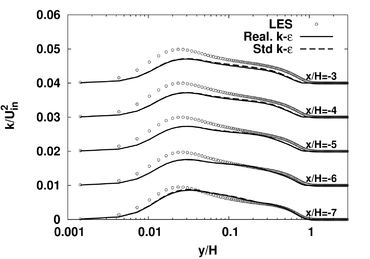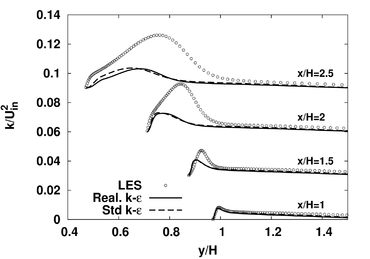(a) (b)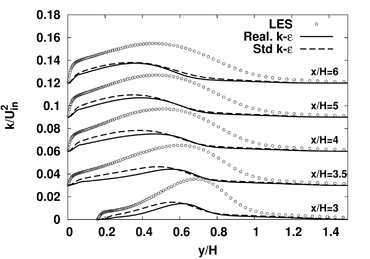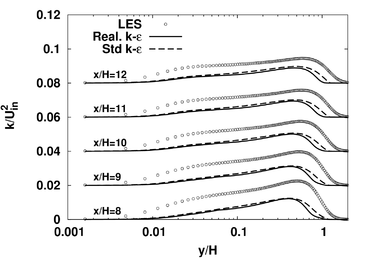(c) (d)

 Figure 10: Comparison of velocity profiles with simulation data for the two ${k-\omega }$models.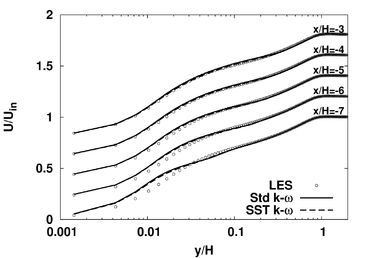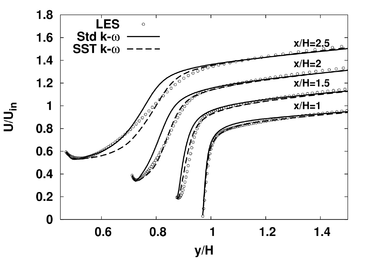(a) (b)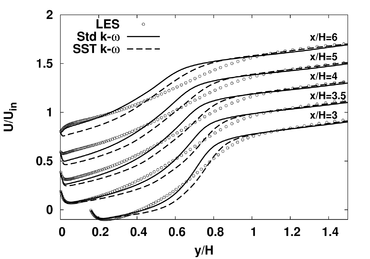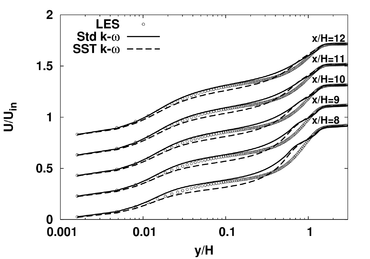(c) (d)

For the ${\ k-\omega }$models, there is a mismatch between the simulated and computed velocity profile immediately downstream of the inlet. The flow is accelerating in the viscous sub-layer (${x/H=-7,\ y/H<0.04}$), while the turbulence profile remains correct. The standard ${\ k-\omega }$formulation notoriously underpredict ${\ k}$in that region, and this overshoot of velocity is due to the imposition of an incompatible turbulence energy. A consistent flow field is only fully established from ${\ x/H=-4}$. Once separation sets in (${\ x/H=1}$), the model returns the same under-prediction of ${\ k}$as the ${\ k-\varepsilon }$variants, both in the separated region and further downstream. Note however that the flow velocity from the Std ${\ k-\omega }$model in the recirculation provides the best agreement with the LES data, with a correct bubble size.

 Figure 11: Comparison of turbulent kinetic energy profiles with simulation data for the two ${k-\omega }$models..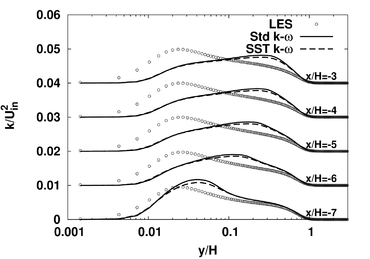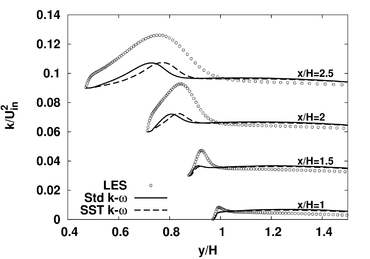(a) (b)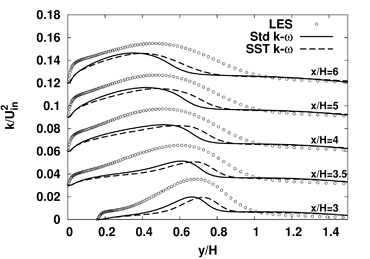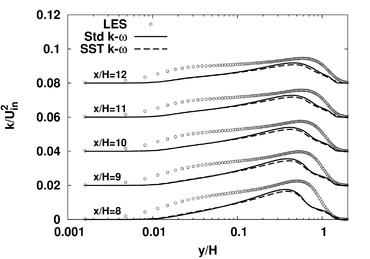(c) (d)

For the most common turbulence models, the results are found to be consistent with previous studies: ${\ k-\varepsilon }$tends to predict a too late separation, which in turn affects the prediction of the reattachment. The opposite trend is observed for both ${\ k-\omega }$models. For all cases, the inflow condition is found to be a very influential parameter, and its definition must be done with great care. An alternative approach, not used in the RANS calculations but in the LES, is to perform a precursor simulation using the same turbulence model that will be tested and save all the relevant quantities at the location where the momentum thickness reaches the desired value.

Contributed by: Sylvain Lardeau, London, UK — CD-adapco